Click to Chat

1800-1023-196

+91-120-4616500

CART 0

• 0

MY CART (5)

Use Coupon: CART20 and get 20% off on all online Study Material

ITEM
DETAILS
MRP
DISCOUNT
FINAL PRICE
Total Price: Rs.

There are no items in this cart.
Continue Shopping• Complete Physics Course - Class 11
• OFFERED PRICE: Rs. 2,968
• View Details

```Application of Hess's Law

1. Calculation of enthalpies of formation

There are large number of compounds such as C6H6, CO, C2H6 etc whose direct synthesis from their constituent element is not possible. Their ΔH0f values can be determined indirectly by Hess’s law. e.g. let us consider Hess’s law cycle for CO2 (g) to calculate the ΔH0f of CO(g) which can not determined otherwise.

C(s) + O2 (g) ———> CO(g) + 1/2 O2(g)       ΔH1  = ?

CO(g) +1/2 O2 (g) ———> CO2 (g)               ΔH2  = –283 KJ/mole

C(s) + O2 (g) ———> CO2 (g)                      ΔH1 = –0393 KJ/mole

According to Hess’s law,

ΔH3 = ΔH1 + ΔH2  or ΔH1 = ΔH3 – ΔH2

= –393 – (–283)  => –110 KJ/mole

2. Calculation of standard Enthalpies of reactions

From the knowledge of the standard enthalpies of formation of reactants and products the standard enthalpy of reaction can be calculated using Hess’s law.

According to Hess’s law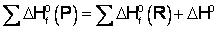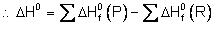ΔHo = [sum of statndard enthalpies of formation of products] – [sum of standard enthalpies of formation of reactants]

3.   In the calculation of bond energies

Bond Energy

Bond energy for any particular type of bond in a compound may be defined as the average amount of energy required to dissociate one mole, viz Avogadro’s number of bonds of that type present in the compound. Bond energy is also called the enthalpy of formation of the bond.

Calculation:

For diatomic molecules like H2, O2, N2, HCl, HF etc, the bond energies are equal to their dissociation energies. For polyatomic molecules, the bond energy of a particular bond is found from the values of the enthalpies of formation. Similarly the bond energies of heteronuclear diatomic molecules like HCl, HF etc can be obtained directly from experiments or may be calculated from the bond energies of homonuclear diatomic molecules.

Sample problem. Calculate the bond energy of HCl. Given that the bond energies of H2 and Cl2 are 430 KJmol-1 and 242 KJ mol-1 respectively and ΔH0f for HCl is -91 KJ mol-1.

Solution:        H2(g) ——> 2H(g)              ΔH = +430 KJmol–1              ...(i)

Cl2(g) ——> 2Cl(g)             ΔH = +242 KJmol–1              ...(ii)

HCl(g) ——> H(g) + Cl(g)    ΔH = ?                                 ...(iii)

For the reaction (iii)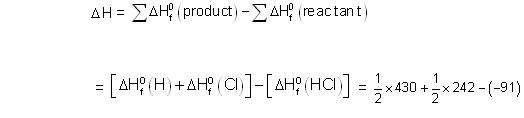ΔH = 427 KJ mol-1

Sample problem.    Given that

2H2(g) + O2(g) ——> H2O(g) , DH = –115.4 kcal the bond energy of H–H and O = O bond respectively is 104 kcal and 119 kcal, then the O–H bond energy in water vapour is

(A) 110.6 kcal / mol                               (B) –110.6 kcal

(C) 105 kcal / mol                                   (D) None

Solution: We know that heat of reaction

ΔH = ΣB.E. (reactant) – ΣB.E (product)

For the reaction,

2H–H(g) + O = O (g) ——> 2H – O–H(g)

ΔH = –115.4 kcal, B.E. of H–H = 104 kcal

B.E. of O=O = 119 kcal

Since one H2O molecule contains two O–H bonds

–115.4 = (2 × 104) + 119 – 4 (O–H) bond energy

∴ 4 (O–H) bond energy = (2 × 104) + 119+115.4

i.e., O–H bond energy = (2×104) + 119 + 115.4 / 4 = 110.6 kcal mol–1

Hence, (A) is correct.

Sample problem. Given the bond energies of N º N, H – H and N – H bonds are 945, 436 and 391 kJ/mol respectively, the enthalpy of the reaction.

N2(g) + 3H2(g) ——> 2NH3(g) is

(A) – 93kJ                                          (B) 102kJ

(C) 90kJ                                              (D) 105kJ

Solution:             (A)

Exercise.

Estimate the average S – F bond energy in SF6. The values of standard enthalpy of formation of SF6(g), SF(g) and F(g) are 1100, 275 and 80 KJ/mole respectively.

LATTICE ENERGY OF AN IONIC CRYSTAL (BORN–HABER CYCLE)Born - Haber cycle

The change in enthalpy that occurs when 1 mole of a solid crystalline substance is formed from its gaseous ions, is known as Lattice energy.

Step 1: Conversion of metal to gaseous atoms

M(s) ——> M(g) ,                    ΔH1 = sublimation

Step 2: Dissociation of X2 molecules to X atoms

X2(g) ——> 2X (g),                    ΔH2 = Dissociation energy

Step 3: Conversion of gaseous metal atom to metal ions by losing electron

M(g) ——> M+ (g) + e–,            ΔH3 = (Ionization energy)

Step 4: X(g) atoms gain an electron to form X– ions

X(g) + e– ——> X–(g),              ΔH4 = Electron affinity

Step 5: M+ (g) and X– (g) get together and form the crystal lattice

M+ (g) + X– (g) ——> MX(s)      ΔH5 = lattice energy

Applying Hess’s law we get

ΔH1 + 1/2 ΔH2 + ΔH3 + ΔH4 + ΔH5 = ΔHf (MX)

On putting the various known values, we can calculate the lattice energy.

Sample problem: What is the expression of lattice energy (U) of CaBr­2 using BornHaber cycle?

Solution:= S + IE­1 + IE2 + D - 2E.A – UCaBr2

Sample problem: What is the relation between ΔH and ΔE in this reaction?

CH4(g) + 2O2(g)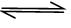CO2(g) + 2H2O(l)

Solution: ΔH = ΔE + ΔnRT

Δn = no. of mole of products - no. of moles of reactants = 1– 3 = –2

ΔH = ΔE – 2RT

Sample problem: What is the expression of lattice energy (U) of CaBr­2?

Using Born Haber cycle?

Solution: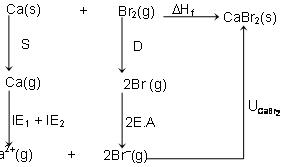ΔHf = S + IE­1 + IE2 + D - 2E.A –

Sample problem: The lattice energy of solid NaCl is 180 kcal/mole. The dissolution of the solid in water in the forms of ions is endothermic to the extent of 1 kcal/mol. If the solution energies of Na+ and Cl– are in the ratio 6:5, what is the enthalpy of hydration of Na+ ion?

(A) - 85.6 kcal/mol                            (B) -97.5 kcal/mol

(C) 82.6 kacl/mol                              (D) +100 kcal/mol

Solution:       (B)

Exercise:

(i) For a system C(s) + O2(g) = CO2(g) which of the following is correct:

(a)  ΔH = ΔE  (b) ΔH >ΔE       (c) ΔH <ΔE (d) ΔH = 0

(ii) C6H6(l) + 15/2 O2(g) —> 3H2O + 6CO2(g) ΔH = –3264.4 kJ mole–1.

What is the energy evolved when 7.8 gm of benzene is burnt in air?

To read more, Buy study materials of Thermodynamics comprising study notes, revision notes, video lectures, previous year solved questions etc. Also browse for more study materials on Chemistry here.
```### Course Features

• 101 Video Lectures
• Revision Notes
• Previous Year Papers
• Mind Map
• Study Planner
• NCERT Solutions
• Discussion Forum
• Test paper with Video Solution